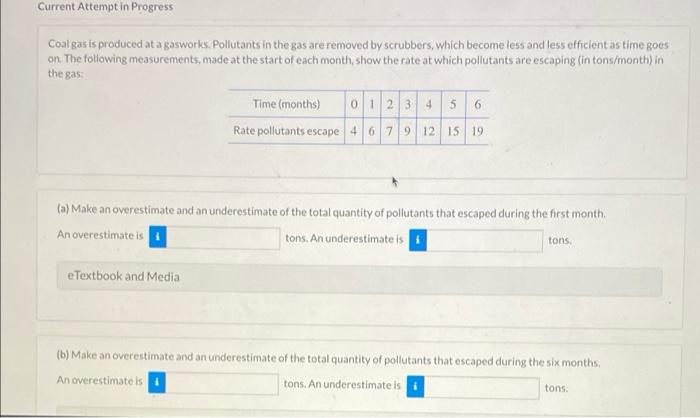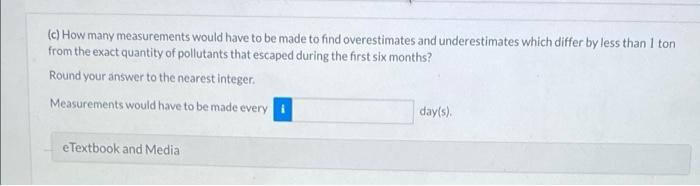# Question Solved1 AnswerCurrent Attempt in Progress Coal gas is produced at a gasworks. Pollutants in the gas are removed by scrubbers, which become less and less efficient as time goes on. The following measurements, made at the start of each month, show the rate at which pollutants are escaping (in tons/month) in the gas Time (months) 0 1 2 3 4 5 6 Rate pollutants escape 4 6 7 9 12 15 19 (a) Make an overestimate and an underestimate of the total quantity of pollutants that escaped during the first month An overestimate is tons. An underestimates tons e Textbook and Media (b) Make an overestimate and an underestimate of the total quantity of pollutants that escaped during the six months An overestimates tons. An underestimate is tons (c) How many measurements would have to be made to find overestimates and underestimates which differ by less than 1 ton from the exact quantity of pollutants that escaped during the first six months? Round your answer to the nearest integer Measurements would have to be made every day(s) e Textbook and MediaTranscribed Image Text: Current Attempt in Progress Coal gas is produced at a gasworks. Pollutants in the gas are removed by scrubbers, which become less and less efficient as time goes on. The following measurements, made at the start of each month, show the rate at which pollutants are escaping (in tons/month) in the gas Time (months) 0 1 2 3 4 5 6 Rate pollutants escape 4 6 7 9 12 15 19 (a) Make an overestimate and an underestimate of the total quantity of pollutants that escaped during the first month An overestimate is tons. An underestimates tons e Textbook and Media (b) Make an overestimate and an underestimate of the total quantity of pollutants that escaped during the six months An overestimates tons. An underestimate is tons (c) How many measurements would have to be made to find overestimates and underestimates which differ by less than 1 ton from the exact quantity of pollutants that escaped during the first six months? Round your answer to the nearest integer Measurements would have to be made every day(s) e Textbook and Media
More
Transcribed Image Text: Current Attempt in Progress Coal gas is produced at a gasworks. Pollutants in the gas are removed by scrubbers, which become less and less efficient as time goes on. The following measurements, made at the start of each month, show the rate at which pollutants are escaping (in tons/month) in the gas Time (months) 0 1 2 3 4 5 6 Rate pollutants escape 4 6 7 9 12 15 19 (a) Make an overestimate and an underestimate of the total quantity of pollutants that escaped during the first month An overestimate is tons. An underestimates tons e Textbook and Media (b) Make an overestimate and an underestimate of the total quantity of pollutants that escaped during the six months An overestimates tons. An underestimate is tons (c) How many measurements would have to be made to find overestimates and underestimates which differ by less than 1 ton from the exact quantity of pollutants that escaped during the first six months? Round your answer to the nearest integer Measurements would have to be made every day(s) e Textbook and Media